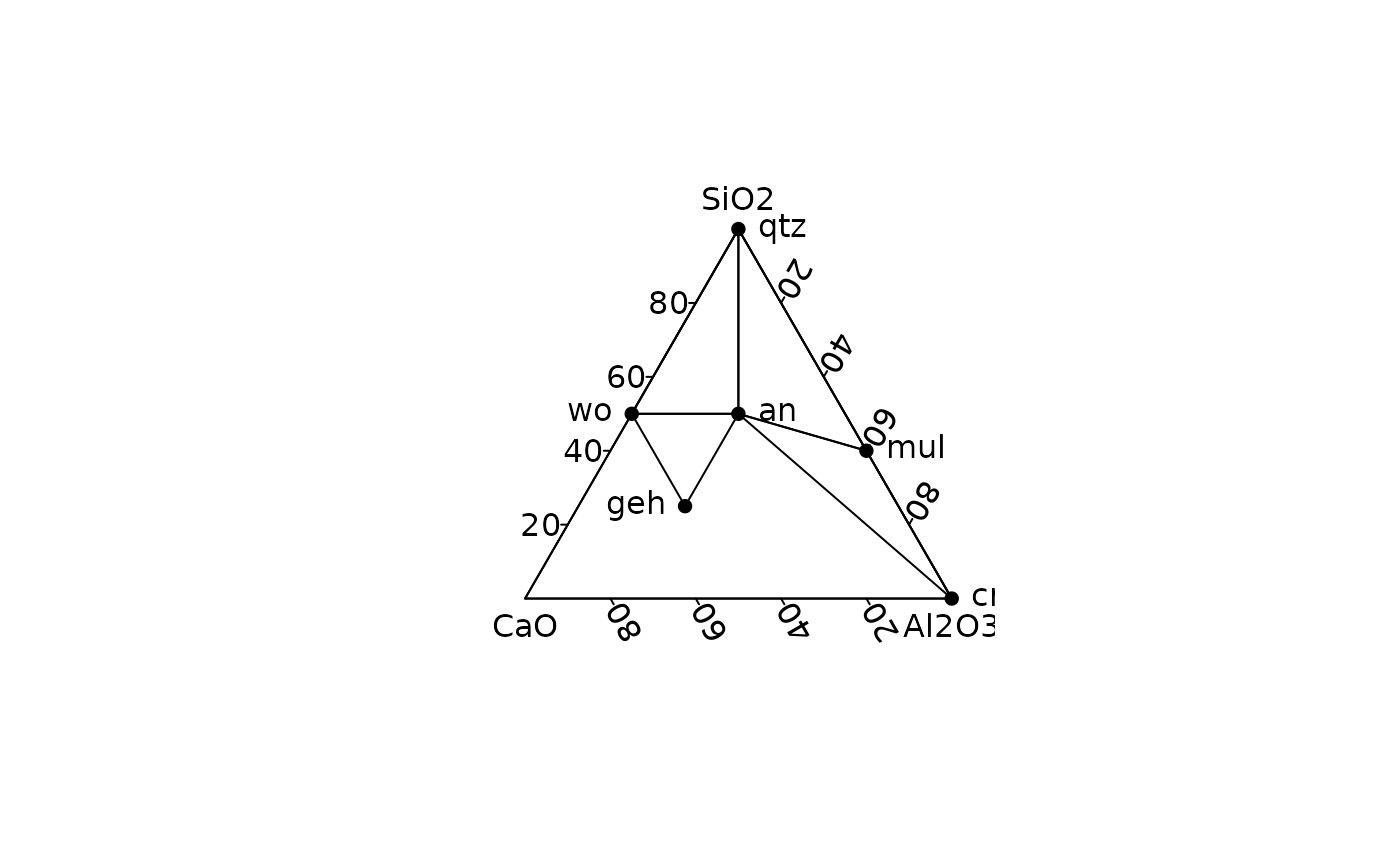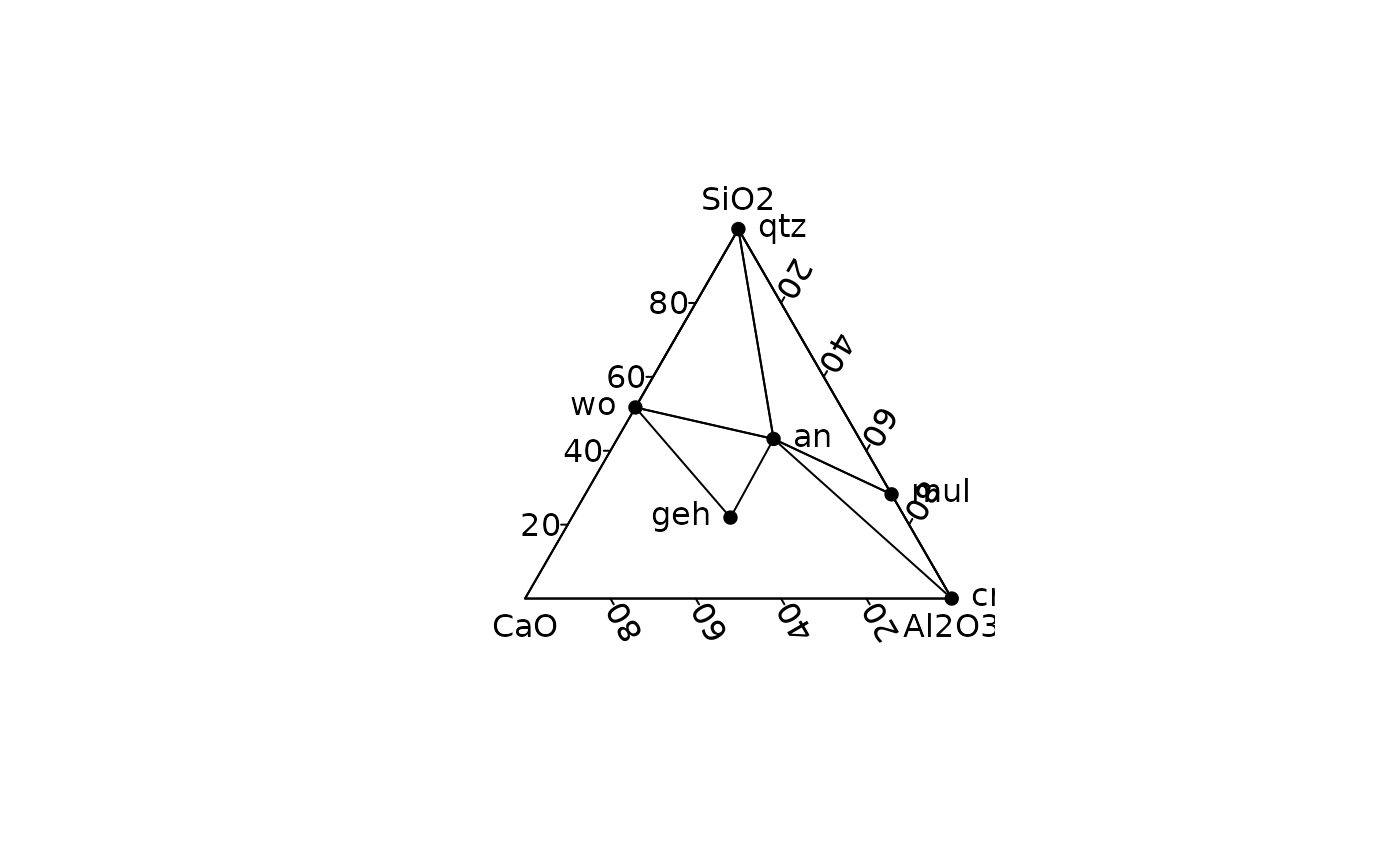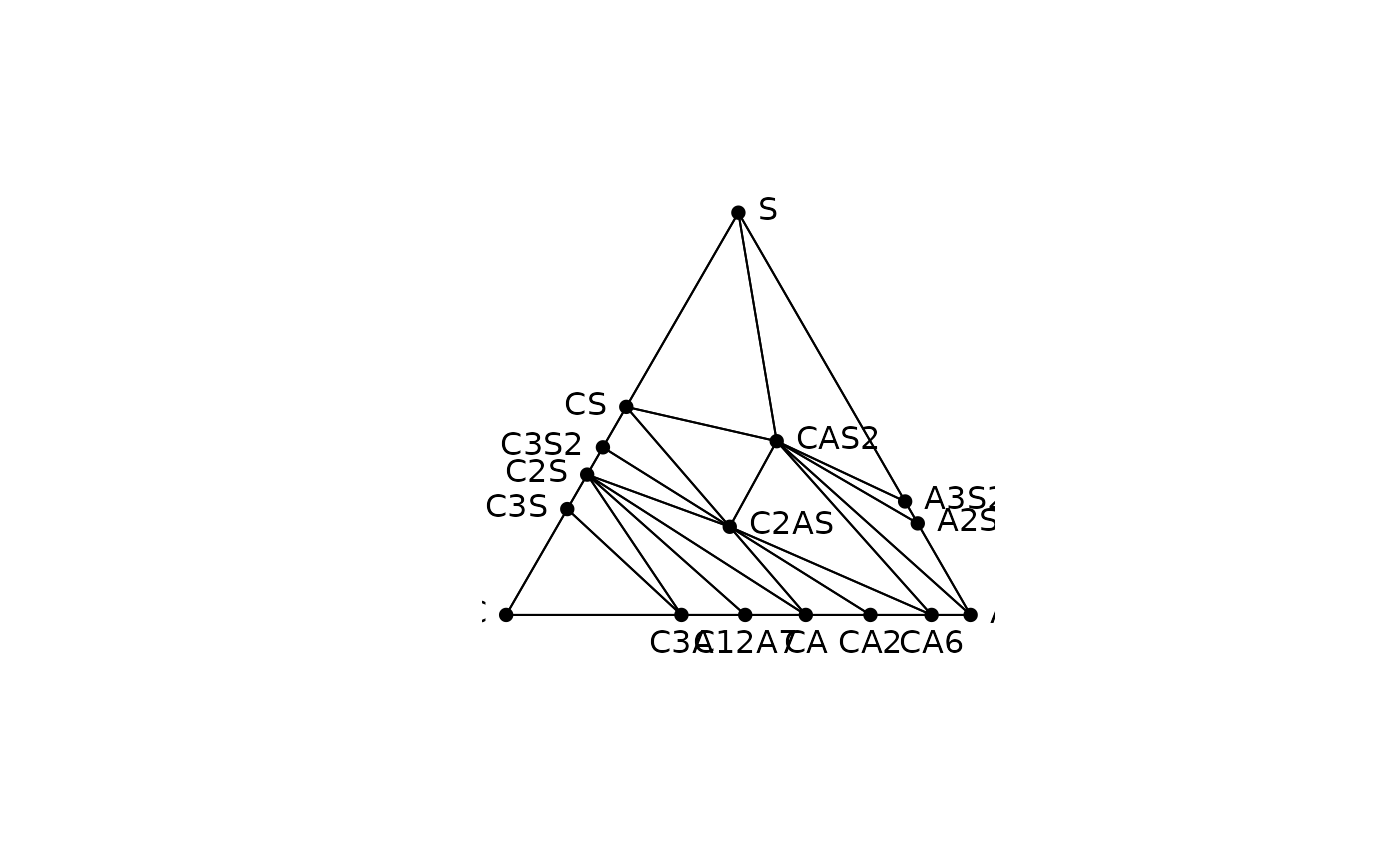Ceramic Phase Diagram

## Usage

triangle_phase_cas(labels = TRUE, symbol = FALSE, mol = FALSE, ...)

triangle_phase_ceramic(labels = TRUE, symbol = FALSE, mol = FALSE, ...)

## Arguments

labels

A logical scalar: should labels be displayed?

symbol

A logical scalar: should symbol be used instead of full labels? Only used if labels is TRUE.

mol

A logical scalar: should molarity be used instead of molar mass?

...

Further arguments to be passed to graphics::polygon().

Other charts: triangle_soil

N. Frerebeau

## Examples

## Ceramic phase diagram
ternary_plot(NULL, xlab = "CaO", ylab = "Al2O3", zlab = "SiO2")
triangle_phase_ceramic(symbol = TRUE, mol = TRUE, pch = 16)ternary_plot(NULL, xlab = "CaO", ylab = "Al2O3", zlab = "SiO2")
triangle_phase_ceramic(symbol = TRUE, mol = FALSE, pch = 16)## CAS diagram
ternary_plot(NULL, axes = FALSE, ann = FALSE, frame.plot = TRUE)
triangle_phase_cas(mol = FALSE, pch = 16)